HOW TO

# Convert slopes with a certain range of slope degree from a DEM file to a polygon feature class in ArcMap

## Summary

A Digital Elevation Model (DEM) file stores elevation data that can be used to extract slope parameters and to perform surface analysis. In some instances, the DEM file is converted to polygons to determine the areas covered by slopes with a certain range of slope degree. For example, areas with slopes ranging from more than 20 degrees are converted to polygons for further analysis and used for development purposes.

For instructions on how to do this in ArcGIS Pro, see: How To: Convert slopes with a certain range of slope degree from a DEM file to a polygon feature class in ArcGIS Pro

## Procedure

These instructions describe how to convert slopes with a certain range of slope degree from a DEM file to a polygon feature class. In this example, slopes with a slope degree of more than 20 degrees are converted to a polygon feature class.

1. In ArcMap, use the Extract by Mask tool to extract the desired region of the study area in the DEM file. For more information, refer to ArcMap Web Help: Extract by Mask.
2. Use the Slope tool from Spatial Analyst Tools > Surface to create the slopes from each cell of the DEM. For more information, refer to ArcMap Web Help: Slope.
3. Change the symbology of the slope map to classify symbology according to the desired range value of the slope degree.
1. Right-click the layer created from the Slope tool and click Properties.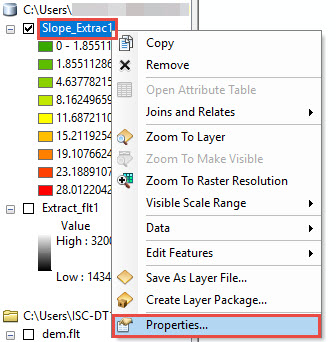2. In the Layer Properties dialog box, click Symbology and select Classified > Classify.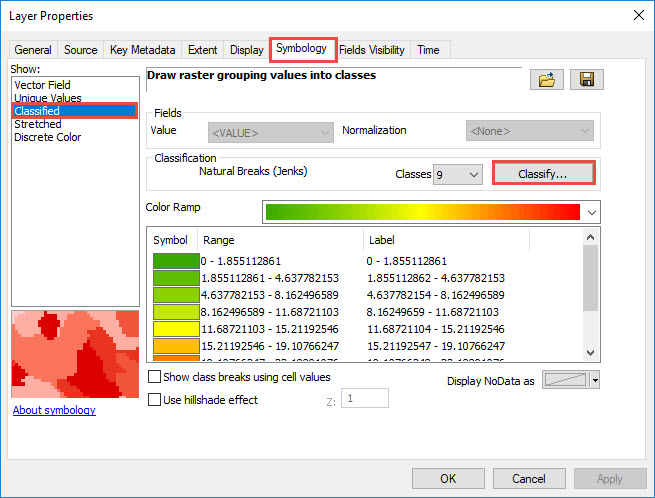3. In the Classification dialog box, select Manual method and set the number of classes. Set the Break Values manually. In this example, the classes are divided into two and the Break Values are set to (0- 20) and ( >20). Click OK.
```Note:
Use the Manual method to define classes. Set class ranges and break values according to the data and the range required. For more information, refer to ArcMap Web Help: Classifying numerical fields for graduated symbology.```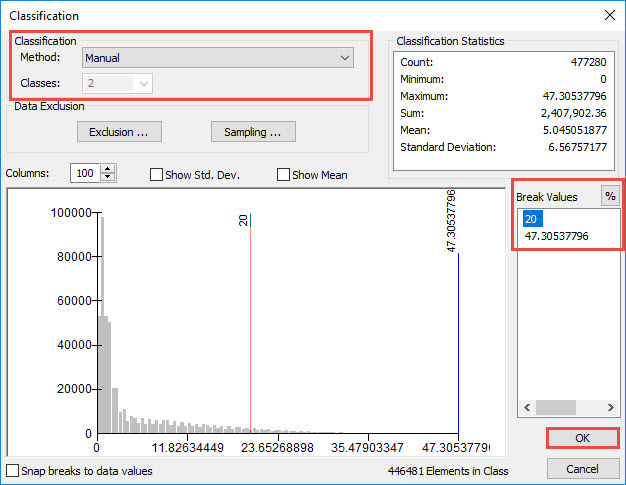1. Click Apply > OK to close the Layer Properties dialog box.
1. Use the Reclassify tool from the Spatial Analyst toolbox to reclassify the range values set for the classified DEM file in Step 3.
1. In the Reclassify dialog box, set the layer in Step 3 as the input raster.
2. Press the Delete key to delete NoData values in the Old Values and New Values column.
```Note:
The New value of 1 represents slopes with the slope degree range of 0 and 20, while new value of 2 represents slopes with the slope degree range of 20 - 47.305378.```
1. Set the output raster layer and click OK.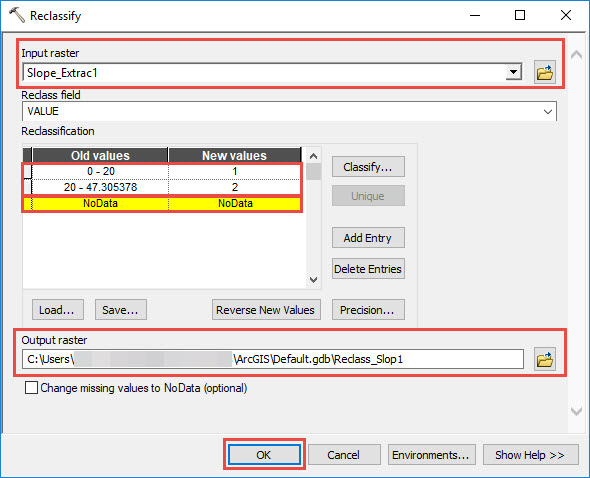1. Use the Raster to Polygon tool from the Conversion toolbox to convert the raster data to a polygon feature class.
1. Open the attribute table of the new layer created from Step 4 and highlight the row to convert to a polygon feature class. For example, to convert the areas with the range of more than 20 degrees, highlight the row with Value 2.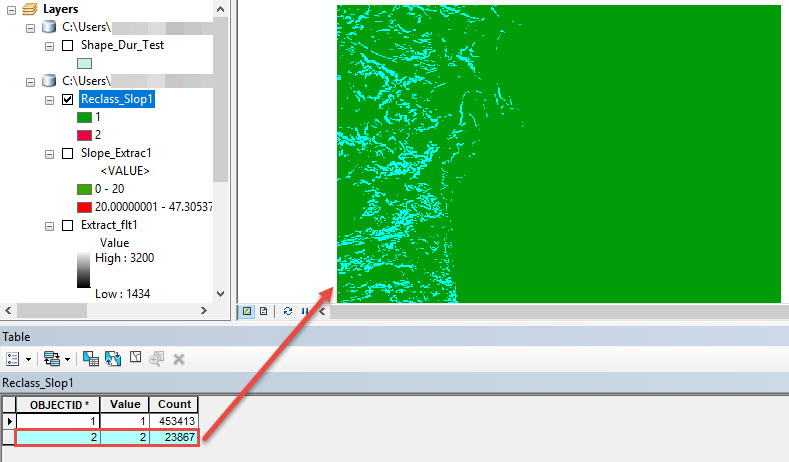2. In the Raster to Polygon dialog box, set the layer from Step 4 as Input raster and set Output polygon features. Click OK.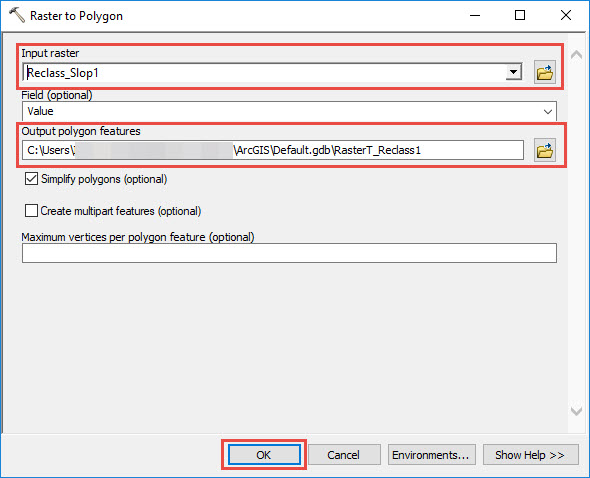A new polygon layer containing the areas of slopes with the slope degree of more than 20 degrees from the Value 2 field is displayed in the map.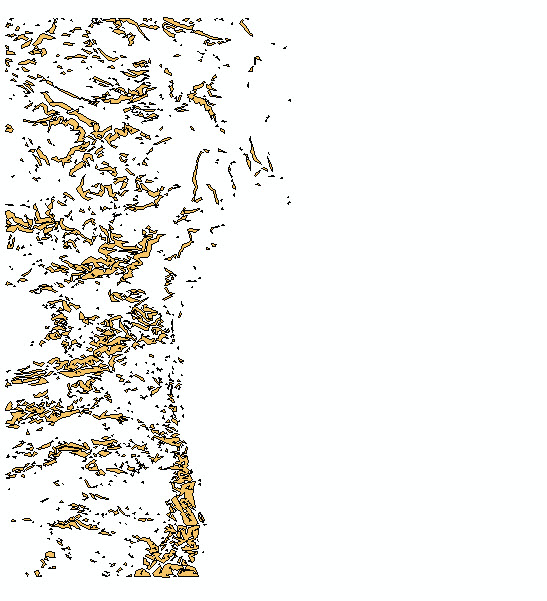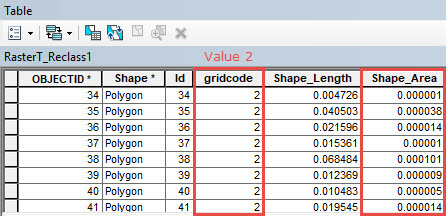Article ID:000019046

Software:
• ArcMap

Get help from ArcGIS experts

Contact technical support